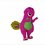# Complex Analysis approach

Hello everyone!

I encountered the following integral a while ago and solved it using Feynman's trick as follows. However, it looks like the residue theorem would be elegant for this problem. Does anyone know how that might work out?

P.S: It would be helpful if someone would check my solution as well.

$I(a,b) = \int_0^{\pi/2} \ln( a \sin^2\theta + b\cos^2\theta)\: d\theta$ Upon taking the partial derivatives wrt $a$ and $b$, we have the following: $\frac{\partial I}{\partial a} = \int_0^{\pi/2} \frac{\sin^2\theta}{a\sin^2\theta + b \cos^2\theta}d \theta, \:\:\:\: \frac{\partial I}{\partial b} = \int_0^{\pi/2} \frac{\cos^2\theta}{a\sin^2\theta + b \cos^2\theta}d \theta$ Next, observe that linear combinations of these two integrals results in integrals that are relatively simple to evaluate. In particular, $a\frac{\partial I}{\partial a} + b\frac{\partial I}{\partial b} = \int_0^{\pi/2} \frac{a\sin^2\theta + b \cos^2\theta}{a\sin^2\theta + b\cos^2\theta}d\theta = \int_0^{\pi/2} d\theta = \frac{\pi}{2}$ and upon summing the two partial derivatives normally, we have $\frac{\partial I}{\partial a} + \frac{\partial I}{\partial b} = \int_0^{\pi/2} \frac{\sin^2\theta + \cos^2\theta}{a\sin^2\theta + b\cos^2\theta}d\theta = \int_0^{\pi/2} \frac{d\theta }{a\sin^2\theta + b\cos^2\theta} = \int_0^{\pi/2} \frac{\sec^2\theta \: d\theta}{b + a\tan^2\theta}$ In order to convert this integral into the more familiar inverse tangent form, we set $\tan\theta = \sqrt{\frac{b}{a}} \tan\varphi$ so that $\sec^2\theta \: d\theta = \sqrt{\frac{b}{a}} \sec^2\varphi \: d\varphi$. For finite $a,b$ and $a\neq 0$, the limits of integration do not change. Then $\frac{\partial I}{\partial a} + \frac{\partial I}{\partial b} = \int_0^{\pi/2} \sqrt{\frac{b}{a}} \cdot \frac{\sec^2\varphi \: d\varphi}{b + a \cdot \frac{b}{a}\tan^2\varphi} = \int_0^{\pi/2} \sqrt{\frac{b}{a}} \cdot \frac{\sec^2\varphi \: d\varphi}{b + a \cdot \frac{b}{a}\tan^2\varphi} = \int_0^{\pi/2} \frac{d\varphi}{\sqrt{ab}}$ $\frac{\partial I}{\partial a} + \frac{\partial I}{\partial b} = \frac{\pi}{2\sqrt{ab}}$ Thus from (1) and (2), we have a system of partial differential equations that can easily be solved. We take $(1) - b \cdot (2)$ to get $(a-b) \frac{\partial I}{\partial a} = \frac{\pi}{2}\bigg( 1- \sqrt{\frac{b}{a}}\bigg), \text{ or equivalently }\:\:\: \frac{\partial I }{\partial a } = \frac{\pi}{2} \cdot \frac{\sqrt{a} - \sqrt{b}}{(a-b)\sqrt{a}} = \frac{\pi}{2\sqrt{a} (\sqrt{a} + \sqrt{b})}$ Upon integrating wrt $a$, we get an analytic solution for $I$, upto a constant $I(a,b) = \int \frac{\pi \: da}{2\sqrt{a}(\sqrt{a} + \sqrt{b})} = \pi \ln(\sqrt{a} + \sqrt{b}) + C$ Notice that this solution is symmetric in $a$ and $b$, just like our system of partial differential equations, so there is no need to integrate wrt $b$. We can differentiate and check that this is indeed a solution to the system of differential equations. To evaluate the constant $C$, notice that for $a=b=1$, we have $I(1,1) = \int_0^{\pi/2} \ln(\sin^2\theta + \cos^2\theta) \: d\theta = 0$ so then for our closed form, we obtain $0 = \pi\ln(2) + C, \text{ or } C = -\pi\ln(2)$ With this, we now have a general solution to this particular family of integrals, $I(a,b) = \pi\ln(\sqrt{a} + \sqrt{b}) - \pi\ln 2 = \pi \ln \frac{\sqrt{a} + \sqrt{b}}{2}$Note by Kishan Jani
6 months, 2 weeks ago

This discussion board is a place to discuss our Daily Challenges and the math and science related to those challenges. Explanations are more than just a solution — they should explain the steps and thinking strategies that you used to obtain the solution. Comments should further the discussion of math and science.

When posting on Brilliant:

• Use the emojis to react to an explanation, whether you're congratulating a job well done , or just really confused .
• Ask specific questions about the challenge or the steps in somebody's explanation. Well-posed questions can add a lot to the discussion, but posting "I don't understand!" doesn't help anyone.
• Try to contribute something new to the discussion, whether it is an extension, generalization or other idea related to the challenge.

MarkdownAppears as
*italics* or _italics_ italics
**bold** or __bold__ bold
- bulleted- list
• bulleted
• list
1. numbered2. list
1. numbered
2. list
Note: you must add a full line of space before and after lists for them to show up correctly
paragraph 1paragraph 2

paragraph 1

paragraph 2

[example link](https://brilliant.org)example link
> This is a quote
This is a quote
    # I indented these lines
# 4 spaces, and now they show
# up as a code block.

print "hello world"
# I indented these lines
# 4 spaces, and now they show
# up as a code block.

print "hello world"
MathAppears as
Remember to wrap math in $$ ... $$ or $ ... $ to ensure proper formatting.
2 \times 3 $2 \times 3$
2^{34} $2^{34}$
a_{i-1} $a_{i-1}$
\frac{2}{3} $\frac{2}{3}$
\sqrt{2} $\sqrt{2}$
\sum_{i=1}^3 $\sum_{i=1}^3$
\sin \theta $\sin \theta$
\boxed{123} $\boxed{123}$

Sort by:

Here is a video by a really good math Youtuber that uses a nice approach also : https://www.youtube.com/watch?v=NAWZx77ZOpw&t=584s

Also, your solution looks good to me :)

- 5 months ago

Thank you so much for the reference! :)

- 5 months ago

I have derived using series manipulation, you may wish to check out here. Also you can find that second solution follows what you have requested.

- 3 months, 2 weeks ago

How did you learn all this at 17? I only recently started learning the basics on real analysis.

- 6 months, 2 weeks ago